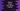# Python numpy floor_divide method explanation with examples## Python numpy floordivide method, Learn to use np.floordivide:

The numpy floor_divide method returns the largest integer value that is smaller or equal to the division of the input items. In this post, we will learn how to use numpy.floor_divide with examples.

### Definition of floor_divide:

The floor_divide method is defined as like below:

``floor_divide(x1, x2, out=None, where=True, casting='same_kind', order='K', dtype=None)``

This method returns the largest integer that is smaller than or equal to the division of the inputs. This is equal to the // operator of Python and it also pairs with the Python %.

### Parameters of floor_divide:

x1 : This is an array_like parameter. It is the numerator. x2 : This is also an array_like parameter. It is the denominator. out: It is the place to store the result. It is an optional parameter. If it is given, it should have the same shape as the input parameters. If this is not provided or if None is passed, it will create a new array with the results and it returns that array. where: It is an optional value, array_like. This is broadcasted over the input array. If it is True for some places, the out array will be set to the result of ufunc. Else, the out array will hold the original value.

### Examples on floor_divide:

Let’s try floor_divide with different examples:

``````import numpy as np

print(np.floor_divide(10, 2))
print(np.floor_divide(5, 2))``````

It will print:

``````5
2``````

Let’s try it with an array and a number:

``````import numpy as np

print(np.floor_divide([10, 20, 30, 40, 50], 2))``````

It will return an array:

``[ 5 10 15 20 25]``

Another example:

``````import numpy as np

print(np.floor_divide([10, 20, 30, 40, 50], 3.45))``````

It will print:

``[ 2.  5.  8. 11. 14.]``